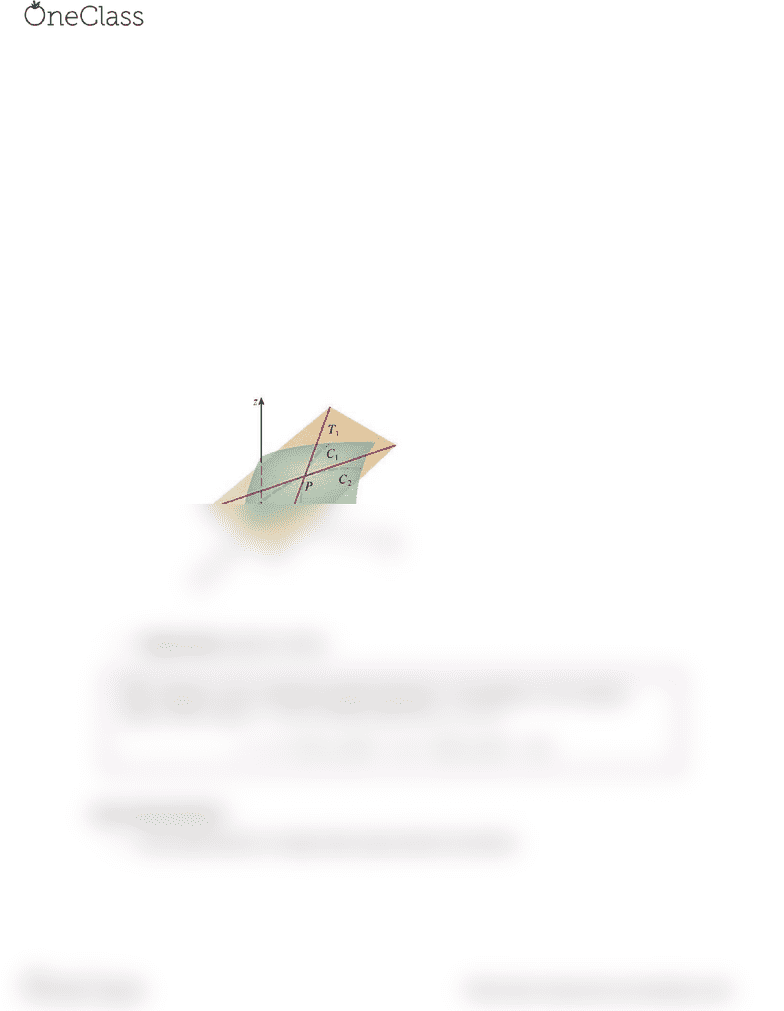Textbook Notes (270,000)
US (100,000)
MATH (40)
Chapter 11.4

MATH 2400 Chapter Notes - Chapter 11.4: Linear Approximation, Differentiable Function, Tangent Space

Department
Mathematics
Course Code
MATH 2400
Professor
Markus Steindl
Chapter
11.4

This preview shows half of the first page. to view the full 2 pages of the document.Anna Pyle
MATH2400 Calculus 3
Textbook: CALCULUS concepts & contexts 4 James Stewart
Tangent Planes and Linear Approximations
Tangent Planes
oThe tangent plane to the surface S at the point P is defined to be the plane that contains
both tangent lines T1 and T2
oThe tangent line S at P consists of all possible tangent lines at P to curves that lie on S
and pass through P
oTangent plane equation is below
Linear Approximations
oLinear approximation or tangent plane approximation (see below)
find more resources at oneclass.com
find more resources at oneclass.com